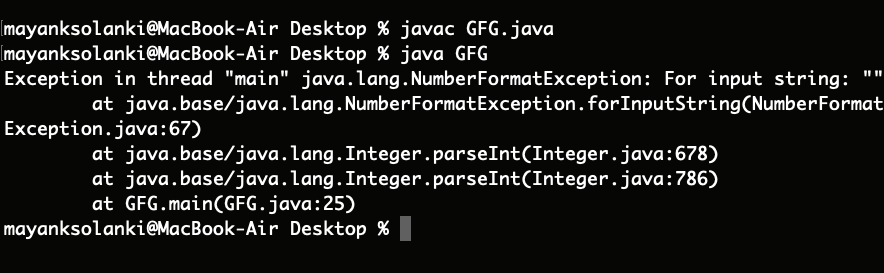Open in App
Not now

# Java Program to Convert a String to Int

• Difficulty Level : Easy
• Last Updated : 07 Jun, 2022

In Java, while operating upon strings, there are times when we need to convert a number represented as a string into an integer type. We usually do this because we can operate with a wide flexible set of operations over strings. The method generally used to convert String to Integer in Java is parseInt() of String class.

### Variants of parseInt() Method

There are two variants of this method:

1. parseInt(String s): This function parses the string argument as a signed decimal integer.
2. parseInt(String s, int radix): This function parses the string argument as a signed integer in the radix specified by the second argument.

Syntax:

`public static int parseInt(String s) throws NumberFormatException`
`public static int parseInt(String s, int radix) throws NumberFormatException`

Parameters:

• A string that needs to be converted to an integer. It can also have the first character as a minus sign ‘-‘ (‘\u002D’) or plus sign ‘+’ (‘\u002B’) to represent the sign of the number.
• The radix is used while the string is being parsed.

Note: This parameter is only specific to the second variant of the method.

Return Type: Both methods convert the string into its integer equivalent. The only difference being is that of the parameter radix. The first method can be considered as an equivalent of the second method with radix = 10 (Decimal).

Note: If your String has leading zeroes, the parseInt() method will ignore them.

Exception Thrown: NumberFormatException is thrown by this method if any of the following situations occur.

Remember certain key points associated with both the variants:

1. The string is null or of zero-length
2. The value represented by the string is not a value of type int
3. Specifically for the parseInt(String s, int radix) variant of the function:
• Any character of the string is not a digit of the specified radix, except that the first character may be a minus sign ‘-‘ (‘\u002D’) or plus sign ‘+’ (‘\u002B’) provided that the string is longer than length 1

Let’s take a random code snippet further understand the illustrations better.

```String str=”0111”;
int t=Integer.parseInt(str);
System.out.println(t); ```

Output:

`111`

For the above code, the snippet output is 111. So, in the cases where leading zeroes are important(in binary representations) avoid using the parseInt() method.

Illustrations:

```parseInt("20") returns 20
parseInt("+20") returns 20
parseInt("-20") returns -20```
```parseInt("20", 16) returns 16, (2)*16^1 + (0)*16^0 = 32
parseInt("2147483648", 10) throws a NumberFormatException```
```parseInt("99", 8) throws a NumberFormatException
as 9 is not an accepted digit of octal number system```
`parseInt("geeks", 28) throws a NumberFormatException`
```parseInt("geeks", 29) returns 11670324,
Number system with base 29 can have digits 0-9
followed by characters a,b,c... upto s```
```parseInt("geeksforgeeks", 29) throws a NumberFormatException as the
result is not an integer.```

### How to use parseInt() Method?

Let us take an example straightaway in order to get used so do the working of parseint() method and implementing the above mentioned as follows:

Example 1:

## Java

 `// Java program to demonstrate working parseInt()` `// Where No NumberFormatException is Thrown`   `// Main class` `public` `class` `GFG {`   `    ``// Main driver method` `    ``public` `static` `void` `main(String args[])` `    ``{` `        ``// Custom wide-varied inputs to illustrate` `        ``// usage of valueOf() method` `        ``int` `decimalExample = Integer.parseInt(``"20"``);` `        ``int` `signedPositiveExample = Integer.parseInt(``"+20"``);` `        ``int` `signedNegativeExample = Integer.parseInt(``"-20"``);` `        ``int` `radixExample = Integer.parseInt(``"20"``, ``16``);` `        ``int` `stringExample = Integer.parseInt(``"geeks"``, ``29``);`   `        ``// Print commands on console` `        ``System.out.println(decimalExample);` `        ``System.out.println(signedPositiveExample);` `        ``System.out.println(signedNegativeExample);` `        ``System.out.println(radixExample);` `        ``System.out.println(stringExample);` `    ``}` `}`

Output

```20
20
-20
32
11670324```

Example 2:

## Java

 `// Java Program to Demonstrate Working of parseInt() Method ` `// Where NumberFormatException is Thrown`   `// Main class` `public` `class` `GFG {`   `    ``// Main driver method` `    ``public` `static` `void` `main(String args[])` `    ``{` `        ``// Custom wide-varied inputs to illustrate` `        ``// usage of valueOf() method ` `        ``int` `decimalExample = Integer.parseInt(``"20"``);` `        ``int` `signedPositiveExample = Integer.parseInt(``"+20"``);` `        ``int` `signedNegativeExample = Integer.parseInt(``"-20"``);` `        ``int` `radixExample = Integer.parseInt(``"20"``, ``16``);` `        ``int` `stringExample = Integer.parseInt(``"geeks"``, ``29``);`   `        ``// It will raise NumberFormatException` `        ``String invalidArguments = ``""``;` `        ``int` `emptyString` `            ``= Integer.parseInt(invalidArguments);` `        ``int` `outOfRangeOfInteger` `            ``= Integer.parseInt(``"geeksforgeeks"``, ``29``);` `        ``int` `domainOfNumberSystem` `            ``= Integer.parseInt(``"geeks"``, ``28``);`   `        ``// Print commands on console` `        ``System.out.println(decimalExample);` `        ``System.out.println(signedPositiveExample);` `        ``System.out.println(signedNegativeExample);` `        ``System.out.println(radixExample);` `        ``System.out.println(stringExample);` `    ``}` `}`

Output:Similarly, we can convert the string to any other primitive data type:

1. parseLong(): parses the string to Long
2. parseDouble(): parses the string to Double
If we want to convert the string to integer without using parseInt(), we can use valueOf() method. It also has two variants similar to parseInt()
3. Difference between parseInt() and valueOf(): parseInt() parses the string and returns the primitive integer type. However, valueOf() returns an Integer object.

Note: valueOf() uses parseInt() internally to convert to integer.

Example 3:

## Java

 `// Java Program to Demonstrate Working of valueOf() Method`   `// Main class ` `public` `class` `GFG {` `  `  `    ``// Main driver method  ` `    ``public` `static` `void` `main(String args[])` `    ``{` `        ``// Custom wide-varied inputs to illustrate` `        ``// usage of valueOf() method ` `        ``int` `decimalExample = Integer.valueOf(``"20"``);` `        ``int` `signedPositiveExample = Integer.valueOf(``"+20"``);` `        ``int` `signedNegativeExample = Integer.valueOf(``"-20"``);` `        ``int` `radixExample = Integer.valueOf(``"20"``, ``16``);` `        ``int` `stringExample = Integer.valueOf(``"geeks"``, ``29``);`   `        ``// Print statements ` `        ``System.out.println(decimalExample);` `        ``System.out.println(signedPositiveExample);` `        ``System.out.println(signedNegativeExample);` `        ``System.out.println(radixExample);` `        ``System.out.println(stringExample);` `    ``}` `}`

Output

```20
20
-20
32
11670324```